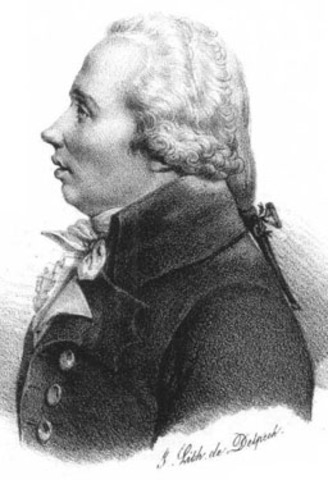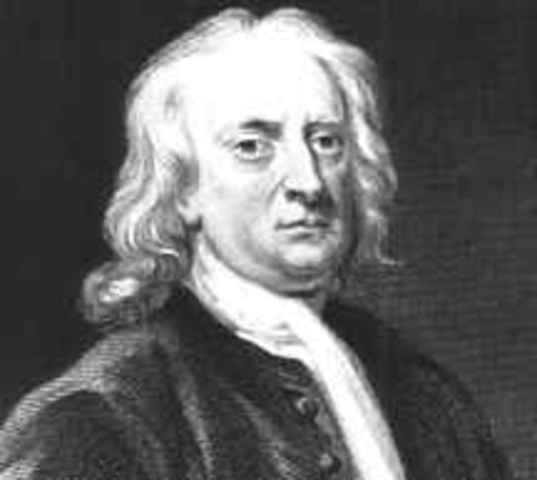• 234

BC. Archimedes• 500

BC. Pythagorus of SamosDeveloped they pythagorean theorem.
A^2 + B^2 = C^2
• Oct 14, 1208

Leonardo `Fibonacci'He introduced the decimal system and the Fibonacci sequence (1,1,2,3,5,8,13...).
• René DescartesDescartes established the Cartesian plane.
• Blaise Pascal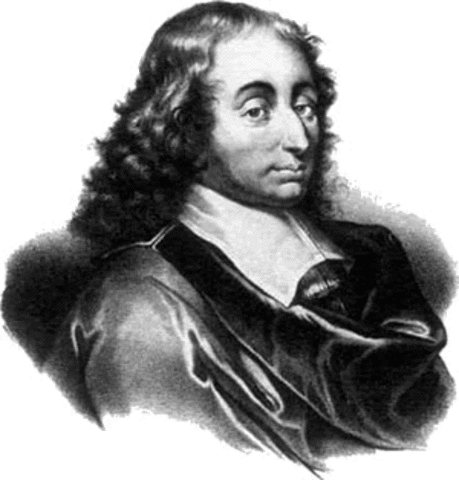He invented one of hte first mechanical calculators. He developed Pascal's triangle.
• Pierre de Fermat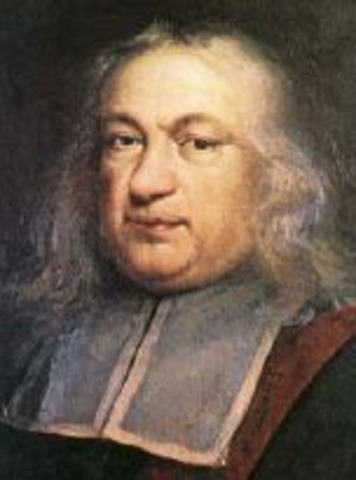He found that every prime number must satisfy, namely 2^(2n) + I = a prime.
• Leonhard Euler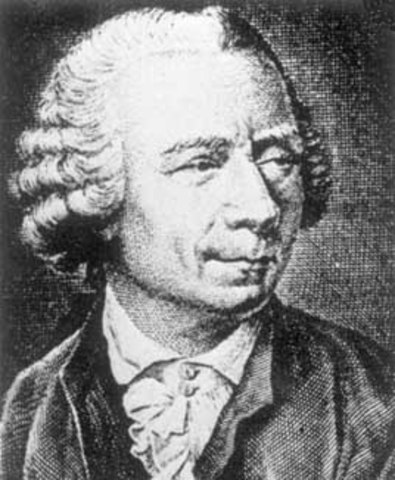Four of the most important constant symbols in mathematics (π, e, i = √-1, and γ = 0.57721566...) were all introduced or popularized by Euler.